NCERT Solutions for Class 7 Maths Chapter 2 Fractions and Decimals Ex 2.4 are part of NCERT Solutions for Class 7 Maths. Here we have given NCERT Solutions for Class 7 Maths Chapter 2 Fractions and Decimals Ex 2.4.

 Board CBSE Textbook NCERT Class Class 7 Subject Maths Chapter Chapter 2 Chapter Name Fractions and Decimals Exercise Ex 2.4 Number of Questions Solved 4 Category NCERT Solutions

## NCERT Solutions for Class 7 Maths Chapter 2 Fractions and Decimals Ex 2.4

Question 1.
Find:Solution: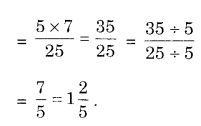Question 2.
Find the reciprocal of each of the following fractions. Classify the reciprocals as proper fractions, improper fractions and whole numbers.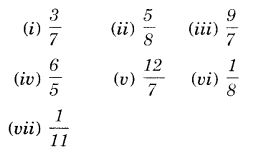Solution: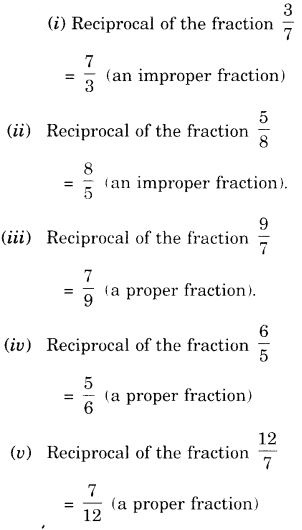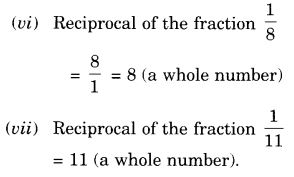Question 3.
Find: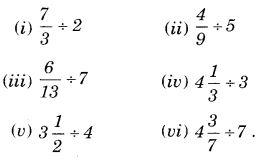Solution:Question 4.
Find: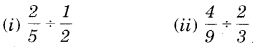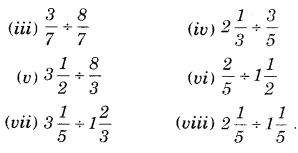Solution:We hope the NCERT Solutions for Class 7 Maths Chapter 2 Fractions and Decimals Ex 2.4 help you. If you have any query regarding NCERT Solutions for Class 7 Maths Chapter 2 Fractions and Decimals Ex 2.4, drop a comment below and we will get back to you at the earliest.